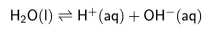# IMAT 2015 Q43 [Pure Water]

Pure water self­-ionises. This endothermic reaction is represented by the equation:Which of the following statements is true for pure water between the temperatures of 0 °C and 100 °C?

1. The concentrations of H^+ (aq) and OH^– (aq) are equal between 0 °C and 100 °C.
2. An increase in temperature causes the pH to fall.
3. An increase in temperature causes the electrical conductivity to decrease.

A. 1 and 3 only
B. 3 only
C. 1 only
D. 1 and 2 only
E. 2 only